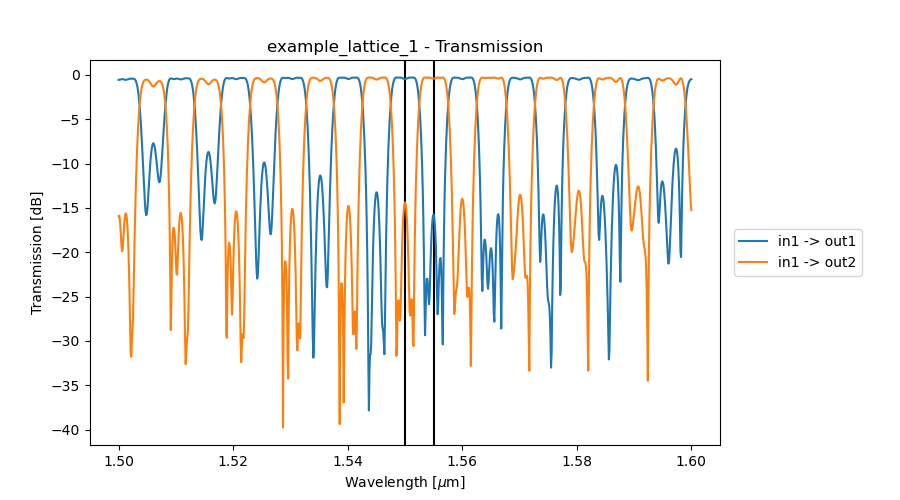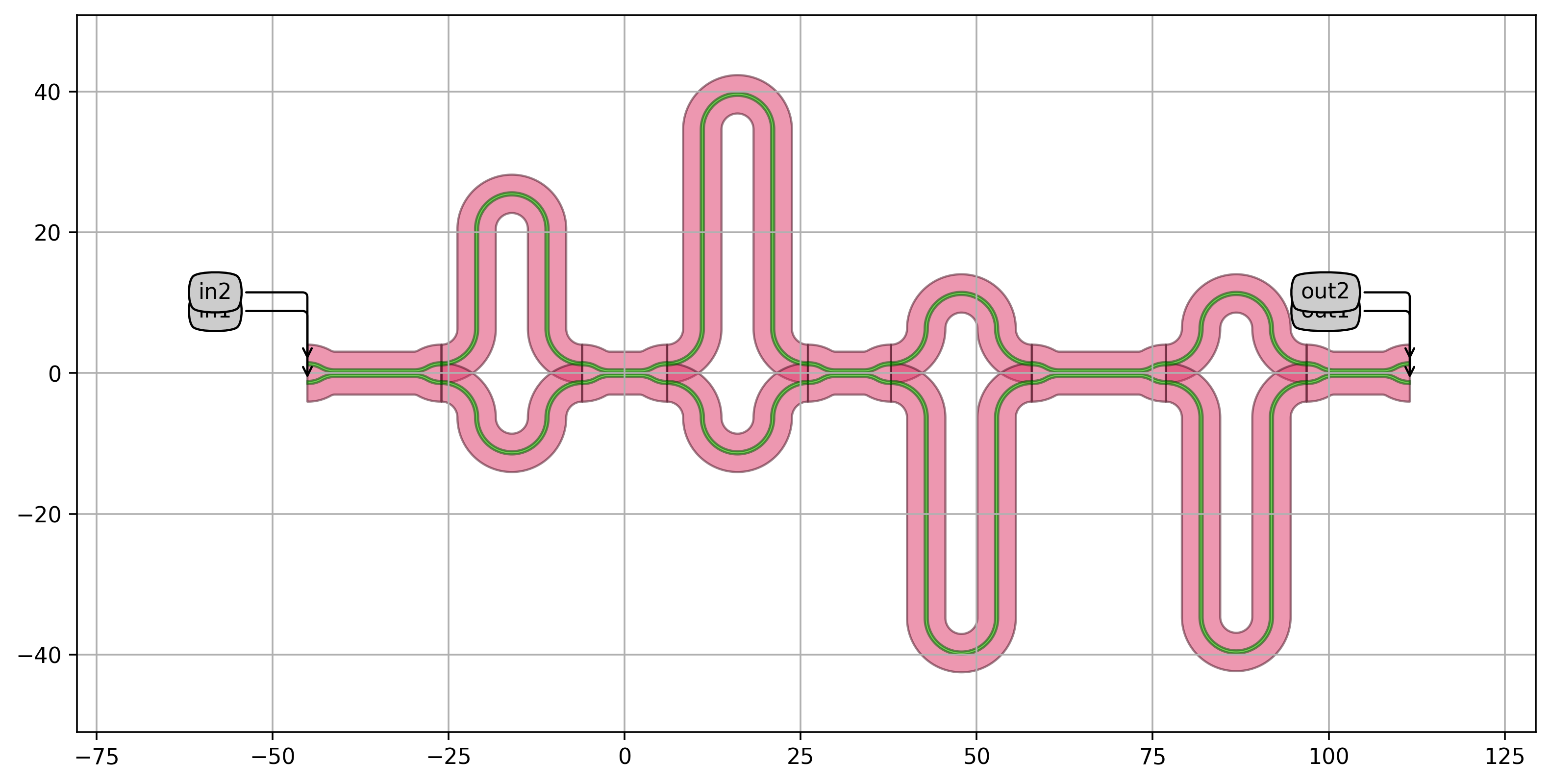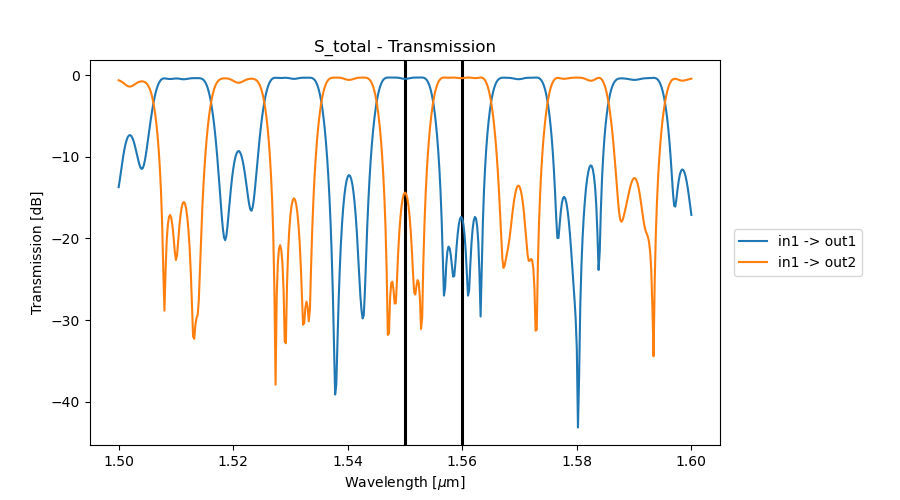# 1.2. CWDM based on cascaded MZI lattice filters¶

In this section, we will explain how to combine MZI lattice filters to obtain demultiplexers with the desired number of stages and channels.

## 1.2.1. Designing our first lattice filter¶

In the following code we use a series of power couplings and delay lengths to design and layout a 2x2 flatband demultiplexer with a certain FSR and center frequency. In the first step, we use the power couplings from this paper (Dwivedi et al. “Coarse Wavelength Division Multiplexer on Silicon-On-Insulator for 100 GbE,” 2015 GFP, pp. 9-10, doi: 10.1109/Group4.2015.7305928.) which were chosen to achieve a maximally flat response.

The delay lengths are calculated from the effective index ($$n_{eff}$$), the group index ($$n_{g}$$), the center wavelength ($$\lambda_{center}$$) and the chosen FSR.

With the help of the following formulas:

$$L = \dfrac{\lambda _{center} ^2}{n_g FSR}$$

embedded in the helper function get_mzi_delta_length_from_fsr and

$$L_{\pi} = \dfrac{\lambda _{center}}{2 n_eff}$$

embedded in the helper function get_length_pi

we can calculate the delay lengths, which are $$[L$$, $$2 L$$, $$(- 2 L + L_{\pi})$$, $$- 2 L]$$.

# Free Spectral Range
fsr = 0.01
# Center Wavelength
center_wavelength = 1.55

# Directional coupler class
coupler_class = pdk.DirectionalCouplerSPower
trace_template = coupler_class().get_default_view(i3.LayoutView).ports.trace_template.cell

# Get the length from the FSR
length = get_mzi_delta_length_from_fsr(trace_template=trace_template,
fsr=fsr,
center_wavelength=center_wavelength)

# Get delta pi
length_pi = get_length_pi(center_wavelength=center_wavelength, trace_template=trace_template)

# Coupling coefficients and delay lengths
power_couplings = [0.5, 0.13, 0.12, 0.5, 0.25]
delay_lengths = [length, 2 * length, -(2 * length + length_pi), -2 * length]

dcs = []
for p in power_couplings:
dc = coupler_class(target_wavelength=center_wavelength, power_fraction=p)
dcs.append(dc)


In the next step, we use the MZILatticeFilter class to generate a lattice filter based on the calculated DC’s and delay lengths and write the result to gds.

# Creating the lattice filter and writing to gds
mzi_lattice = MZILatticeFilter(directional_couplers=dcs,
delay_lengths=delay_lengths)

mzi_lattice_lv = mzi_lattice.Layout()
mzi_lattice_lv.visualize(annotate=True)
mzi_lattice_lv.write_gdsii("lattice_example1.gds")


Finally, we simulate the lattice filter.

# Simulation of the lattice filter
cell_cm = mzi_lattice.CircuitModel()
wavelengths = np.linspace(1.5, 1.6, 501)
S = cell_cm.get_smatrix(wavelengths=wavelengths)
channels = [center_wavelength + cnt * fsr / 2 for cnt in range(2)]
name = "example_lattice_1"
save_dir = os.path.join(os.path.dirname(os.path.realpath(__file__)), "_images", name)
if not os.path.exists(save_dir):
os.makedirs(save_dir)
fig = plt.figure()
for p in range(2):
plt.plot(wavelengths, 10 * np.log10(np.abs(S["in1", "out{}".format(p + 1)]) ** 2), '-',
label="{}_in1_out{}".format(name, p + 1), linewidth=2.2)
for x in channels:
plt.axvline(x=x, linewidth=2.2, color='black')
plt.xlabel("Wavelengths [um]", fontsize=16)
plt.ylabel("Transmission [dB]", fontsize=16)
plt.title("{} - Transmission".format(name), fontsize=18)
plt.legend(fontsize=14, loc='center left', bbox_to_anchor=(1, 0.5))
plt.xlim(1.5, 1.6)
plt.ylim((-50, 2))
plt.show()
fig.savefig(os.path.join(save_dir, "S_total.png"), bbox_inches='tight')
plt.close(fig)## 1.2.2. Implementing the lattice filter as a class¶

Once the implementation strategy is chosen, we can directly inherit from the MZI lattice filter defined in the previous section to design a two-way wavelength demultiplexer. We define a Python class that inherits from MZILatticeFilter to create a demultiplexer with 50% of the given free spectral range (FSR) at the specified center wavelength.

class Mux2(MZILatticeFilter):
"""Two-way wavelength demultiplexer with a passband of 50% of the FSR at the center_wavelength.
This component inherits from MZILatticeFilter.
Power couplings are taken from 'Coarse Wavelength Division Multiplexer on Silicon-On-Insulator
for 100 GbE': DOI:10.1109/group4.2015.7305928
"""
power_couplings = i3.LockedProperty(default=[0.5, 0.13, 0.12, 0.5, 0.25])
delay_lengths = i3.LockedProperty()
fsr = i3.PositiveNumberProperty(default=0.01, doc="Free spectral range of the MUX2")
center_wavelength = i3.PositiveNumberProperty(default=1.55, doc="Center wavelength")

def _default_directional_couplers(self):
dir_couplers = [pdk.DirectionalCouplerSPower(name=self.name + "dc_{}".format(cnt),
power_fraction=p,
target_wavelength=self.center_wavelength)
for cnt, p in enumerate(self.power_couplings)]
return dir_couplers

def _default_delay_lengths(self):

tt = self.directional_couplers.get_default_view(i3.LayoutView).ports.trace_template.cell
length = get_mzi_delta_length_from_fsr(center_wavelength=self.center_wavelength,
fsr=self.fsr,
trace_template=tt)

length_pi = get_length_pi(center_wavelength=self.center_wavelength,
trace_template=tt)

delay_lengths = [length, 2 * length, -(2 * length + length_pi), -2 * length]
return delay_lengths

return 5.0


The properties of this class are:

• power_couplings: this is a locked property.
• delay_lengths: this is a locked property.
• bend_radius: this is a locked property. Its default value is 5 um.
• fsr: this property is not locked and can be changed when instantiating the PCell. The default value is 0.01.
• center_wavelength: this property is not locked. The default value is 1.55 um.

We can now instantiate this PCell and simulate it:

1. Instantiate the component, its layout and visualize it.

    # Writing the layout
cell = Mux2(name="MUX2",
fsr=0.02,
center_wavelength=1.55)
cell_lv = cell.Layout()
cell_lv.visualize(annotate=True)
cell_lv.write_gdsii("mux2.gds")2. Instantiate and simulate the circuit.

    # Simulating the circuit
wavelengths = np.linspace(1.5, 1.6, 501)
cell_cm = cell.CircuitModel()
S = cell_cm.get_smatrix(wavelengths=wavelengths)

3. Plot the transmission between the input port in1 and the two output ports out1 and out2.